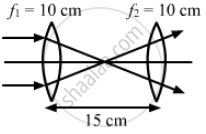Department of Pre-University Education, KarnatakaPUC Karnataka Science Class 12
Advertisement Remove all ads

# Two Convex Lenses, Each of Focal Length 10 Cm, Are Placed at a Separation of 15 Cm with Their Principal Axes Coinciding. (A) Show that a Light Beam Coming Parallel to the Principal Axis Diverges - Physics

Sum

Two convex lenses, each of focal length 10 cm, are placed at a separation of 15 cm with their principal axes coinciding. (a) Show that a light beam coming parallel to the principal axis diverges as it comes out of the lens system. (b) Find the location of the virtual image formed by the lens system of an object placed far away. (c) Find the focal length of the equivalent lens. (Note that the sign of the focal length is positive although the lens system actually diverges a parallel beam incident on it.)

Advertisement Remove all ads

#### Solution

Given,
Focal length of each convex lens, f = 10 cm
Distance between both the lens, d = 15 cm(a) As shown in the figure, the light rays are falling parallel to the principal axis of the first lens, therefore the rays will converge within the focal length of the second lens. Hence, all the rays emerging from the lens system are diverging.
(b) Using lens formula for first convex lens,
$\frac{1}{v} = \frac{1}{f} + \frac{1}{u}$
$\Rightarrow \frac{1}{v}=\frac{1}{10}+\frac{1}{( - \infty )}$
$\Rightarrow \frac{1}{v}=\frac{1}{10}$
$\Rightarrow v = 10 \text{ cm }$
For the second convex lens,
Object distance, u = −(15 − 10) = −5 cm
If v1 is the image distance for the second convex lens, then,
Applying lens formula we have:
$\frac{1}{v_1} = \frac{1}{f} + \frac{1}{u}$
$\Rightarrow \frac{1}{v_1} = \frac{1}{10} + \frac{1}{- 5}$
$\Rightarrow \frac{1}{v_1} = \frac{- 1}{10}$
$\Rightarrow v_1 = - 10 cm$

Thus, the virtual image of the object will be at 5 cm from the first convex lens.

(c) The equivalent focal length of the lens system
$\left( F \right)$ is given by,
$\frac{1}{F} = \frac{1}{f_1} + \frac{1}{f_2} - \frac{d}{f_1 f_2}$
$Here, f = f_1 = f_2 = 10 \text{ cm }$
$\therefore \frac{1}{F} = \frac{1}{10} + \frac{1}{10} - \frac{15}{100}$
$\Rightarrow \frac{1}{F} = \frac{5}{100}$
$\Rightarrow F = 20 \text{ cm }$

Therefore the equivalent focal length (F) is 20 cm...

Concept: Refraction at Spherical Surfaces and by Lenses - Refraction at Spherical Surfaces
Is there an error in this question or solution?
Advertisement Remove all ads

#### APPEARS IN

HC Verma Class 11, 12 Concepts of Physics 1
Chapter 18 Geometrical Optics
Q 72 | Page 416
Advertisement Remove all ads

#### Video TutorialsVIEW ALL 

Advertisement Remove all ads
Share
Notifications

View all notifications

Forgot password?